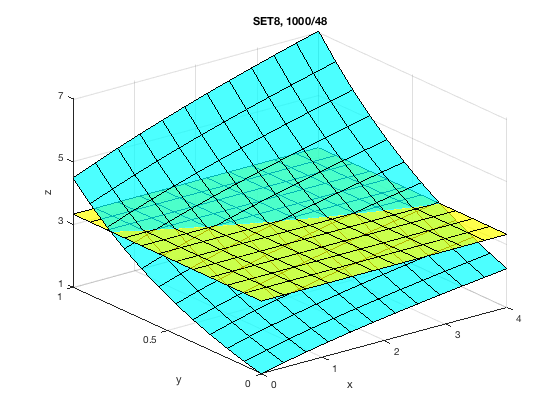# MATH 251: Calculus 3, SET8

## 15: Multiple Integrals

### 15.1: Double Integrals Over Rectangles

These problems are done with the CAS. See Hand Solutions for details.

#### 1. [999/10]

With a rectangular region of integration, we can always easily change the order of integration since the limits of integration are constants. It may be the case that an integral is harder (or even impossible) to integrate analytically (by hand or with a CAS) in a particular order. So be open to changing the order of integation if needed.
syms x y
Ixy = int(int(2*x+1, x, 0, 2), y, 0, 4) % The result
Ixy = 24
Iyx = int(int(2*x+1, y, 0, 4), x, 0, 2) % is the same.
Iyx = 24

#### 2. [999/14]

syms x y
f(x,y) = y*sqrt(x+2)
f(x, y) =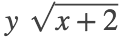I1 = expand( int(f(x,y), x, 0, 2) )
I1 =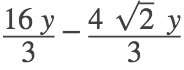I2 = int(f(x,y), y, 0, 3)
I2 =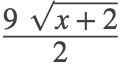#### 3. [1000/18]

Here we additionally approximate the result after computing the exact value.
syms x y
I = expand( int(int(sin(x) + sin(y), y, 0, pi/2), x, 0, pi/6) )
I =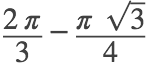I_appx = double(I)
I_appx = 0.7340

#### 4. [1000/22]

An approximate answer is often useful in scientific and engineering work.
I = int(int(y*exp(x-y), x, 0, 2), y, 0, 1)
I =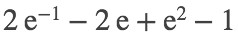I_appx = double(I)
I_appx = 1.6883

#### 5. [1000/26]

syms s t
I = int(int(sqrt(s+t), s, 0, 1), t, 0, 1)
I =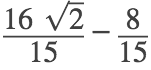I_appx = double(I)
I_appx = 0.9752

#### 6. [1000/30]

syms t theta
I = int(int(tan(theta) / sqrt(1-t^2), t, 0, 1/2), theta, 0, pi/3)
I =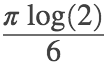I_appx = double(I)
I_appx = 0.3629

#### 7. [1000/34]

syms x y
I = int(int(1/(1+x+y), x, 1, 3), y, 1, 2)
I =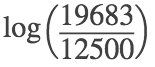I_appx = double(I)
I_appx = 0.4540

#### 8. [1000/40]

syms x y
z1 = x^2 + x*y^2, z2 = 0 % Curved surface is above xy-plane (z=0).
z1 =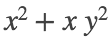z2 = 0
V = int(int(z1-z2, x, 0, 5), y, -2, 2)
V =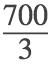V_appx = double(V) % in cm^3
V_appx = 233.3333

#### 9. [1000/44]

syms x y
z1 = 2*x*y / (x^2+1), z2 = x + 2*y
z1 =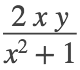z2 =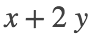figure
fsurf(z1, [0 2 0 4], 'g', 'MeshDensity', 12); hold on
fsurf(z2, [0 2 0 4], 'r', 'MeshDensity', 12)
view(130,20); xlabel('x'); ylabel('y'); zlabel('z')
xticks(0:2); yticks(0:2:4); zticks(0:2:10)
title('SET8, 1000/44: Plane is above curved surface.')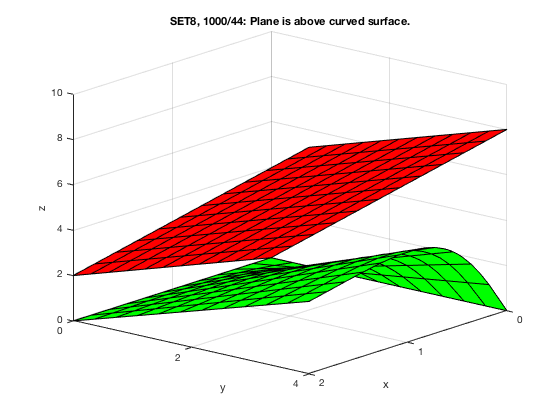%
V = int(int(z2-z1, x, 0, 2), y, 0, 4)
V =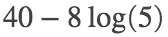V_appx = double(V) % in cm^3
V_appx = 27.1245

#### 10. [1000/48]

The average value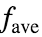of f over a region R is given by.
• In Calculus 1, the region of integration was an interval. Hence the measure is the length of the interval.
• For a double integral in Calculus 3, the measure of a 2D region is its area.
• For a triple integral in Calculus 3, the measure of a 3D region is its volume.
syms x y
f(x,y) = exp(y)*sqrt(x+exp(y))
f(x, y) =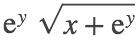I = int(int(f(x,y), y, 0, 1), x, 0, 4)
I =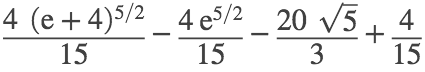A = 4*1
A = 4
f_ave = I/A
f_ave =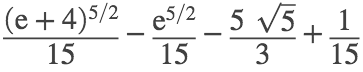f_ave_appx = double(f_ave)
f_ave_appx = 3.3270
%
figure
fsurf(f(x,y), [0 4 0 1], 'c', 'MeshDensity', 12); hold on
fsurf(f_ave, [0 4 0 1], 'y', 'MeshDensity', 12)
xlabel('x'); ylabel('y'); zlabel('z'); alpha 0.7
xticks(0:4); yticks(0:0.5:1); zticks(1:2:7)
title('SET8, 1000/48') % The average value represents the average height of the surface.